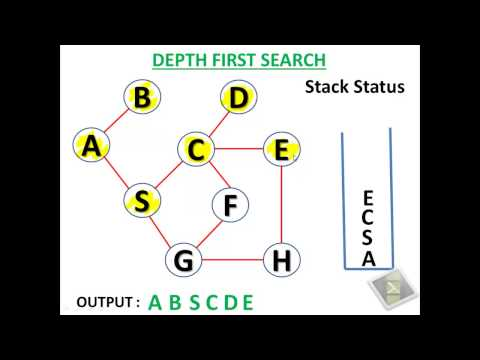# Implementing DFS (Depth First Search) for Graph Traversal in PythonDFS is another very common graph traversal and searching algorithm. DFS uses backtracking to traverse the nodes in a graph. DFS tries to go far away from the start node until it reaches a dead node or leaf node. Then it uses backtrack to get back until it has new node to visit. Unlike BFS which uses a queue, DFS uses stack data structure to keep track of the traversed nodes.

Here is an youtube video which explains the DFS with a nice visual example:Here is a sample graph which we will use to test our DFS algorithm implemented in Python.### DFS using iterative function call

``````def dfs(graph, start):
visited = [start]
stack = [start]

while len(stack) > 0:
current = stack[-1]

# Checking the all the adjacent nodes of current node has been visited or not.
# If yes, then remove the current node from the stack
if set(graph[current]).issubset(set(visited)):
stack.pop()
continue

for node in graph[current]:
if node in visited:
continue

stack.append(node)
visited.append(node)
break

return visited

if __name__ == '__main__':
graph = {
1: [1, 2, 5],
2: [3, 5],
3: [2, 4],
4: [3, 5, 6],
5: [1, 2, 4],
6: 
}

paths = dfs(graph, 1)

print(paths)
``````

### DFS using recursive function call

Recursive functions use stacks to keep track of the execution status known as execution stack. We can implement the DFS using recursive functions which will naturally use this execution stack as the stack we needed to implement in the above iterative implementation to keep track of our processing nodes in graph. Here is the recursive implementation of DFS without using any stack:

``````def dfs(graph, start, visited=[]):
if start not in visited:
visited.append(start)

for node in graph[start]:
if node in visited:
continue

visited = dfs(graph, node, visited)

return visited

if __name__ == '__main__':
graph = {
1: [1, 2, 5],
2: [3, 5],
3: [2, 4],
4: [3, 5, 6],
5: [1, 2, 4],
6: 
}

paths = dfs(graph, 1)

print(paths)``````

Here is the output for the travered path:

``[1, 2, 3, 4, 5, 6]``

So, now as we learned how to code a DFS, when to use it or it's other counter part BFS? Here is a good discussion about some practical cases regarding when to use DFS or BFS: